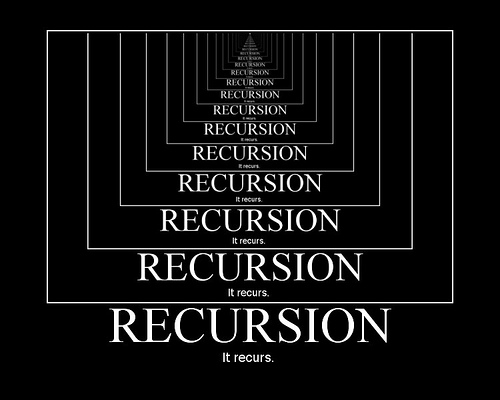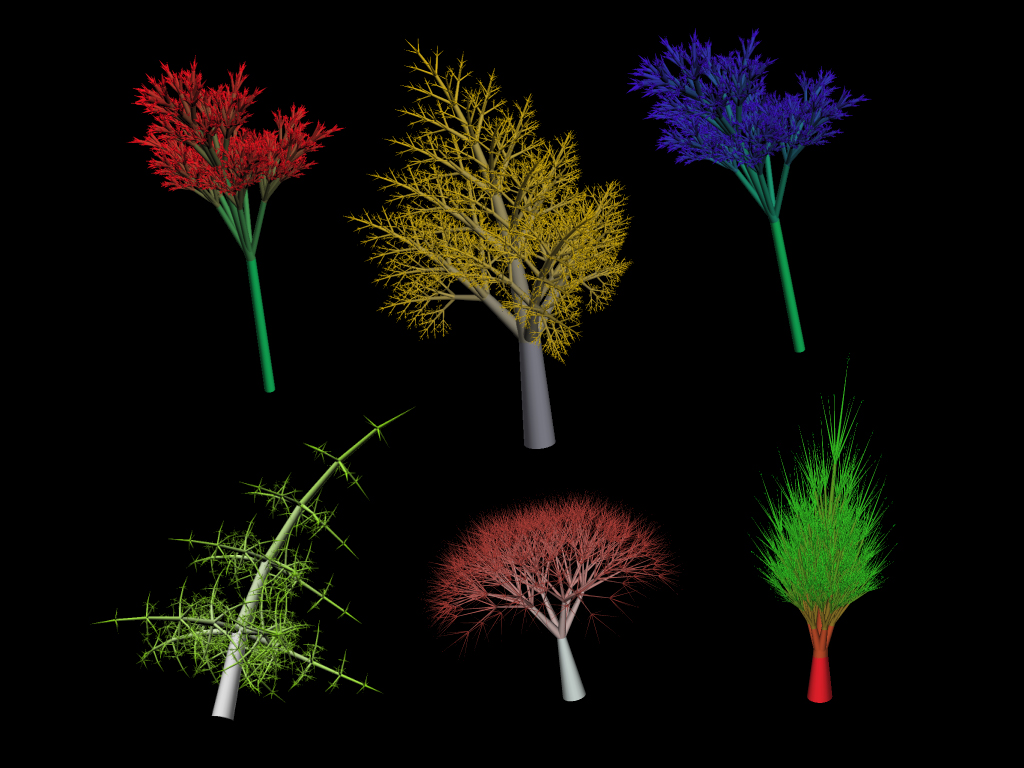# Goals

• Understanding Recursion
• Defining Recursion
• Components of Recursion
• Tail Recursion

# Resources

"To understand recursion, you must first understand recursion." ~ Anon

# Defining Recursion

Recursion is defined as the repeated application of a recursive definition.
A recursive definition is a method who's output for a specific input is based on the output of the same method with related but different inputs.
Some examples from nature:

• A section of a shell spiral is a different sized copy of the section before it
• A tree branch can sprout another branch like the trunk that sprouted it
• A cell divides in two, each copy has the ability to divide in two, like the firstRecursion is based on a recursive definition. It is a way to describe something in terms of itself.

# The Base Case

What if the shell were to keep on spiraling, the tree branches branching, and the cells dividing? It would be chaos! There would be no end to the recursion. That is why we must always apply a base case to our recursive methods in order to get them to stop. Here are some examples of a base case.

if (size < 2) { //stop }
if (size > 2) { //continue } else { //stop }
if (num == 1) { //stop }

A base case is nothing more than an if statement which ensures the recursion will stop at some point.

# Recursive Parameters and Arguments

A recursive method is defined by the parameters it has. The arguments to the parameters usually come from the previous calls to the recursive method. There is of course the initial arguments to the recursive method, and setting this up can be a bit tricky. Take a look at this example which computes the factorial of the argument in the first method call inside setup: